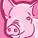# App Development

Announcements
Now Live: Qlik Sense SaaS Simplified Authoring – Analytics Creation for Everyone: READ DETAILS
cancel
Showing results for
Did you mean:Contributor

## Subtract every row by specific row value

Hi,

I have, for example, the following data:

Asset   Performance

Asset 1     5%

Asset 2     3%

Asset 3     8%

Asset 4     10%

I would like to create a table showing every Asset and the result of Asset x Performance - Asset 1 Performance. The result should be the following:

Asset 1  0%

Asset 2  -2%

Asset 3  3%

Asset 4   5%

I wrote Sum([Performance])-sum({<Asset={'Asset 1'}>}[Performance]) and, as a result, only Asset 1 is being subtracted.

Any ideas?

Labels (3)

• ### Set Analysis

1 Solution

Accepted SolutionsMVP

You are missing the TOTAL qualifier from the second expression

``Sum([Performance]) - Sum(TOTAL {<Asset = {'Asset 1'}>}[Performance])``
7 RepliesMVP

You are missing the TOTAL qualifier from the second expression

``Sum([Performance]) - Sum(TOTAL {<Asset = {'Asset 1'}>}[Performance])``Contributor
Author

Thanks Suuny, it worked. Now I have an extra problem. There are Assets that have missing values, no performance instead of 0%. So, following the example, if Asset 5 has no performance, I need to see -5% as a result, but now I see nothing.

Any idea?

Thanks!MVP

Try this

``````RangeSum(
Sum([Performance]),
-Sum(TOTAL {<Asset = {'Asset 1'}>}[Performance])
)``````Contributor
Author

Hi Sunny,

It didn't work, but we can keep on this in other question.

Many thanksMVP

Would you be able to share a sample to show what exactly you are seeing?Contributor
Author

Sorry that I'm not able to show the data. Using the formula above, I see this result:The first row is OK, since it subtracted the Asset 1 - Asset 1=0. But in the rest of the rows, I would need to do the same in all the rows, not only the values that the row has.MVP

Will be difficult to comment without seeing a sample and being able to play around with it.